## Monday, April 30, 2012

### A Basic Intro To Copulas in SAS

What is a copula?
A copula can be defined as a multivariate distribution with marginals that are uniform over the unit interval (0,1).  Copula functions can be used to simulate a dependence structure independently from the marginal distributions.

Based on Sklar's theorem the multivariate distribution F can be represented by copula C as follows:
F(x1…xp) = C{ F1(x1),…, Fp(xp); θ}

where  each Fi(xi) is a uniform marginal distribution and θ  is a dependence parameter. ‘Copula’ means to connect or join. Copula functions in essence connect or join the information in the marginal to form a multivariate distribution.

Why not just use measures of correlationn or multivariable regression to describe relationships between varaibles?

As described by Tang and Valdez

"While being computationally convenient and straightforward to understand, linear correlations fail to capture all the dependence structure that exist between losses from multiple business lines."

Zimmer and Trivedi (2005) also elaborate on how copula methods can be more useful than traditional correlation approaches:

“econometricians often possess more information about marginal distributions of related variables than their joint distribution. The copula approach is a useful method for deriving joint distributions given the marginal distributions, especially when the variables are nonnormal....When fairly general  and/or asymmetric modes of dependence are relevant, such as those that go beyond correlation or linear association, then copulas play a special role in developing additional concepts and measures.”

Dorey and Joubert discuss the importance of copulas in actuarial work:

"The increasing complexity of insurance and reinsurance products has led to interest in the modelling of dependent risks, especially with the emergence of intricate multi-line products.....Extreme events, such as hurricanes, can also result in dependencies between classes of business which are unrelated in normal conditions"

Example

Let’s take a subset of data (from the SAS Online Documentation) for stock returns.

data returns;
input  ret_msft  ret_duk;
cards;
0.004182    0.003503
-0.027960   -0.000582
0.006732    0.025611
-0.033435   -0.002838
0.029560    0.010814
-0.003054   -0.001689
-0.012255   -0.012408
0.013958    0.003427
-0.011318   -0.017075
-0.022587   0.002316
;
run;

The next block of data uses PROC COPULA to estimate the covariance structure of the returns of Microsoft and Duke Energy.

/* Copula estimation */
proc copula data = returns;
var  ret_msft ret_duk;
fit normal / outcopula=estimates;
run;

/* keep only correlation estimates */
data estimates;
set estimates;
keep ret_msft ret_duk;
run;

Next, we can use PROC COPULA to simulate the multivariate distribution of returns based on the correlation estimates derived before, using the Gaussian Copula.

/* Copula simulation */
proc copula;
var ret_msft ret_duk;
define cop normal (cor = estimates);
simulate cop / ndraws     = 500
outuniform = simulated_uniforms
plots=(data=uniform scatter);
run;

The Financial Crisis:  A Case Study for Copula Specifications Using SAS

For an interesting 2 part video visualizing the construction of collateralized debt obligations and tranches see here and here.

From: In defense of the Gaussian copula, The Economist

"The Gaussian copula provided a convenient way to describe a relationship that held under particular conditions. But it was fed data that reflected a period when housing prices were not correlated to the extent that they turned out to be when the housing bubble popped."

Various functional forms for copula functions exist, each based on different assumptions about the dependence structure of the underlying variables.

Using SAS PROC COPULA , and loosely following Zimmer 2012, I have simulated data based on 3 different copula formulations and produced scatter plots for each. Using the table provided in Zimmer, I solved for the dependence parameter θ in each copula formulation giving a dependence structure consistent with Kendall’s tau = .60 (SAS code follows).

A mentioned in the Economist article at the beginning of this post, the Gaussian copula was used widely before the housing crisis to simulate the dependence between housing prices in various geographic areas of the country. Looking at the plot for the Gaussian copula above, it can be seen that extreme events (very high values of Y1 and Y2 or very low values of Y1 and Y2) seem very weakly correlated compared to the plots for the Clayton and Gumbel copulas. We can see that with the Gumbel copula, extreme events (very high values of Y1 and Y2) are more correlated, while with the Clayton copula, extreme events (very low Y1 and Y2) are more correlated.

Recall, as previously stated, linear correlations may provide a measure of dependence, but they fail to capture the complete structure of dependence. For instance, in each of plots above, we get the following measures of the Pearson Correlation Coefficient (.81,  .78, & .79) for the Gaussian, Gumbel, and Clayton copula simulations respectively.  This measure is in essence the same across all three simulations, ~ .80. However, this tells us nothing about the dependence relationship for extreme values of Y1 and Y2. Copulas, if properly specified, help us to better specify the structure of dependence.

How might this relate to the financial crisis specifically?  In The Role of Copulas in the Housing Crisis, Zimmer states:

"Due to its simplicity and familiarity, the Gaussian copula is popular in the calculation of risk in collaterized debt obligations. But the Gaussian copula imposes asymptotic independence such that extreme events appear to be unrelated. This restriction might be innocuous in normal times, but during extreme events, such as the housing crisis, the Gaussian copula might be inappropriate"

In the paper a mixture of Clayton and Gumbel copulas is investigated as an alternative to using the Gaussian copula.

SAS CODE

*-------------------------------------------------------------------------
| theata : kendal's tau = .60
*--------------------------------------------------------------------------;
* gaussian;
data inparm;
input COL1 COL2;
cards;
1 .81
.81 1
;
run;

proc copula ;
var Y1-Y2;
define cop normal (cor=inparm);
simulate cop /
ndraws     = 500
seed       = 1234
outuniform = normal_unifdata
plots      = (data = original scatter
distribution = cdf);
run;

* gumbel ;
proc copula ;
var Y1-Y2;
define cop gumbel (theta=2.5);
simulate cop /
ndraws     = 500
seed       = 1234
outuniform = normal_unifdata
plots      = (data = original scatter
distribution = cdf);
run;

* clayton copula;
proc copula ;
var Y1-Y2;
define cop clayton (theta=3);
simulate cop /
ndraws     = 500
seed       = 1234
outuniform = normal_unifdata
plots      = (data = original scatter
distribution = cdf);
run;

For a related visualization of copulas using excel, see below:

References:

SAS Online Documentation: PROC COPULA

The Role of Copulas in the Housing Crisis. David M. Zimmer.
The Review of Economics and Statistics, May 2012, 94(2): 607–620

Copula Modeling:  An Introduction for Practitioners
Pravin K. Trivedi1 and David M. Zimmer2
Foundations and Trends in Econometrics
Vol. 1, No 1 (2005) 1–111
c 2007 P. K. Trivedi and D. M. Zimmer
DOI: 10.1561/0800000005

Economic Capital and the Aggregation of Risks using Copulas
Andrew Tang and Emiliano A. Valdez†
School of Actuarial Studies
Faculty of Commerce & Economics
University of New South Wales
Sydney, AUSTRALIA

Understanding Relationships Using Copulas
Edward W. Frees† and Emiliano A. Valdez‡
North American Actuarial Journal, Vol. 2, No. 1.
Modelling Copulas: An Overview
Martyn Dorey Phil Joubert
The Staple Inn Actuarial Society

## Saturday, April 21, 2012

### Analysis of GMO vs Non-GMO Corn Hybrid Persistence Using Simulated Time Dependent Covariates in SAS

#### Abstract

Fitting survival models utilizing time dependent covariates with the PHREG procedure in SAS involves the utilization of programming statements within the procedure that involve array processing. Based loosely on the descriptive statistics reported by Ma and Shi, I use the SAS data step loop to simulate a data set consisting of 10,000 corn hybrids including variables for genetic modification, vertical integration, and the number of close substitutes for each hybrid for each time period. This paper demonstrates the use of PROC PHREG to fit a Cox Proportional Hazards model utilizing the simulated time dependent covariates. In addition, I demonstrate the use of the %PUT statement to verify that values of the time dependent covariates are correctly utilized for each period.

FULL TEXT

#### Suggested Citation

Matt Bogard. "Analysis of GMO vs Non-GMO Corn Hybrid Persistence Using Simulated Time Dependent Covariates in SAS" 2012
Available at: http://works.bepress.com/matt_bogard/19

## Thursday, April 19, 2012

### Survival Analysis

Let ‘T’ be a random variable time to event. Then the distribution of ‘T’ can be described as follows: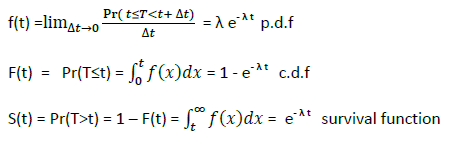The Hazard function is a conditional density function giving the instantaneous risk that an event will occur at time‘t’.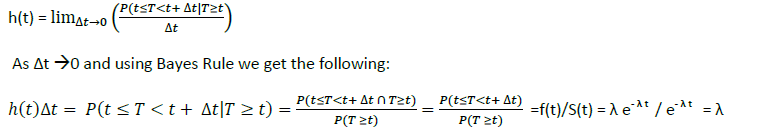Using R, crude plots of f(t), F(t), S(t), and h(t)  for λ = .5 are given below: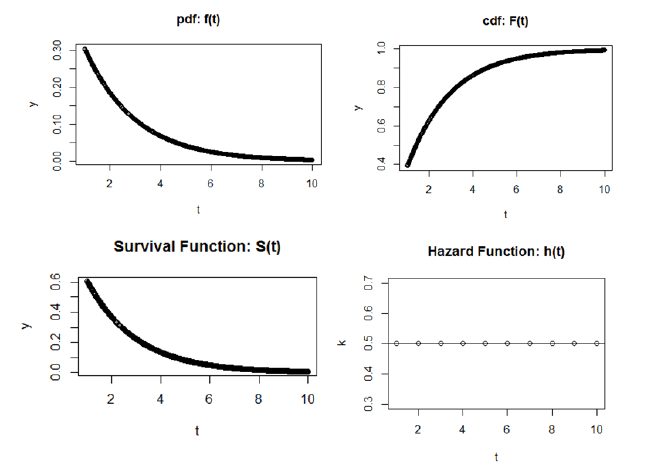If we let h(t) = λ or λ0 or ln h(t) = ln(λ0)  = μ  be the ‘baseline’ hazard,  we can easily extend the model to include covariates as such:

h(t) = λ0(t) exp(β 1X12X2)
ln h(t) = ln[λ0(t) exp(β 1X12X2)]
= ln λ0(t) + ln[exp(β 1X12X2)]
= μ + β 1X12X2
Note: if X1 and X2 = 0 then we get the baseline hazard

Cox Proportional Hazards Model
If we specify the hazard for two individuals i and i’ :

hi(t) = λ0(t) exp(β 1X1i2X2i)
hi’(t) = λ0(t) exp(β 1X1i’2X2i’)

Then the proportional hazard for individual i relative to i’ can be written as:

hi(t)/ hi’(t) = λ0(t) exp(β 1X1i2X2i)/ λ0(t) exp(β 1X1i’2X2i’)
with the ‘baseline’ hazard term cancelling out we get:
hi(t)/ hi’(t) = exp[β 1(X1 i –X1i’)  +β2(X2 i –X2i’) ] or e ζ i- ζ i’

As a result, we don’t have to explicitly know the functional form of the baseline hazard to calculate the proportional hazard.

Partial Likelihood Estimation

The parameters for the proportional hazard model are estimated via partial likelihood estimation.

Introducing a censoring indicator ‘ci’ {=0 if censored at event time else 1} we can express the likelihood function as: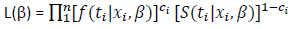Instead of maximizing the full likelihood specified above, Cox proposed estimating the partial likelihood which does not include the baseline hazard function: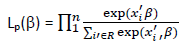The risk set contains subjects at risk for the event at time ‘ti ‘when the event was observed for subject ‘i’. Censoring times are excluded from the likelihood.

Based on the Cox model, hazard was proportional to exp(xi'B) The ratio in the partial likelihood represents the hazard for subject ‘i’ relative to the cumulative hazard for all other subjects at risk at the time subject ‘i’ experienced the event. The estimates for β can be derived by maximizing Lp(β) , and can be interpreted as follows: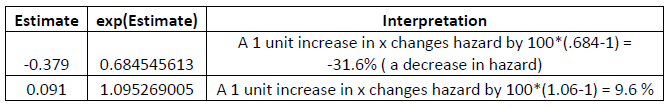References:
Survival Analysis Using SAS: A Practical Guide. Paul D. Allison. 1995. The SAS Institute.
Cox Proportional-Hazards Regression for Survival Data:
Appendix to An R and S-PLUS Companion to Applied Regression. John Fox Februrary 2002

Introduction to Survival Analysis. Sociology 761 Lecture Notes. John Fox. 2006.

Introduction to Survival Analysis. (ppt presentation). Kristin Sainani. Ph.D.  Stanford University. Department of Health Research and Policy. http://www.stanford.edu/~kcobb